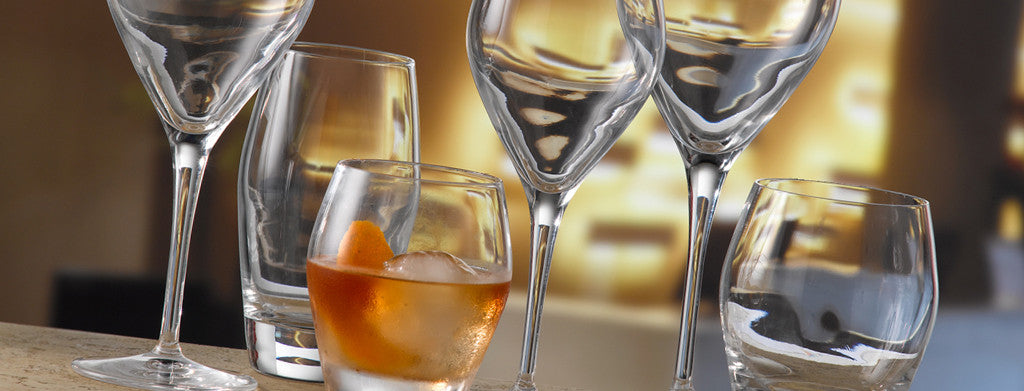Shopping Cart

### Refine

View all

#### Price# Glassware£3.71 each (ex VAT)£1.77 each (ex VAT)£3.42 each (ex VAT)£6.19 each (ex VAT)£7.37 each (ex VAT)£2.30 each (ex VAT)£1.93 each (ex VAT)£1.72 each (ex VAT)£8.59 each (ex VAT)£5.28 each (ex VAT)£4.05 each (ex VAT)£2.68 each (ex VAT)£4.23 each (ex VAT)£2.67 each (ex VAT)£3.42 each (ex VAT)£4.67 each (ex VAT)£5.56 each (ex VAT)£2.07 each (ex VAT)£3.41 each (ex VAT)£3.11 each (ex VAT)£3.69 each (ex VAT)£8.93 each (ex VAT)£6.19 each (ex VAT)£6.13 each (ex VAT)£3.65 each (ex VAT)£3.49 each (ex VAT)£2.02 each (ex VAT)£0.67 each (ex VAT)£4.65 each (ex VAT)£6.13 each (ex VAT)£2.37 each (ex VAT)£2.48 each (ex VAT)£6.80 each (ex VAT)£5.28 each (ex VAT)£3.73 each (ex VAT)£2.30 each (ex VAT)£2.38 each (ex VAT)£2.95 each (ex VAT)£6.28 each (ex VAT)£5.96 each (ex VAT)£5.20 each (ex VAT)£4.82 each (ex VAT)£4.20 each (ex VAT)